Bonky Zhu
If someone is able to show me that what I think or do is not right, I will happily change, for I seek the truth, by which no one was ever truly harmed. It is the person who continues in his self-deception and ignorance who is harmed.

自动图灵测试：评估对话的回答

1. Introduction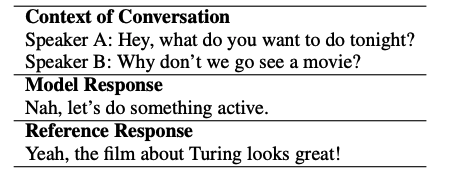ELIZA：一种基于规则的系统，通过不断地改写陈述或提问来模仿罗杰式心理治疗师。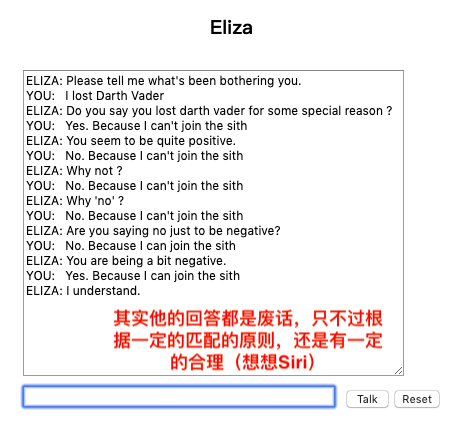2. 数据收集

• 由基于TF-IDF检索的模型选择的回答，
• 由双编码器（DE）选择的回答，
• 使用分层递归编码器/解码器（HRED）模型生成的回答，
• 人为生成的回答。

3. 背景

3.2 Word-Overlap Metrics 关于计算词重叠的方法

BLEU： 分析了回答中n-gram的共同出现次数，用它来计算整个数据集的n-gram精度，然后将其乘以长度惩罚因子以惩罚简短翻译。对于BLEU-N，N表示所考虑的最大ngram值（通常N = 4）。

1. 捕获单词重叠统计信息以外的语义相似性；

ADEM使用分层RNN编码器学习上下文，模型回答和参考回答的分布式表示。给定对话上下文$c$，参考回答$r$和模型回答$\hat r$，ADEM首先使用RNN编码器将它们中的每一个编码为矢量（分别为$c$，$r$和 $\hat r$ ）。然后，ADEM使用线性变换空间中$c$，$r$ 和 $\hat r$ 的矢量表示之间的点积计算分数：
$$\operatorname{score}(c, r, \hat{r})=\left(\mathbf{c}^{T} M \hat{\mathbf{r}}+\mathbf{r}^{T} N \hat{\mathbf{r}}-\alpha\right) / \beta$$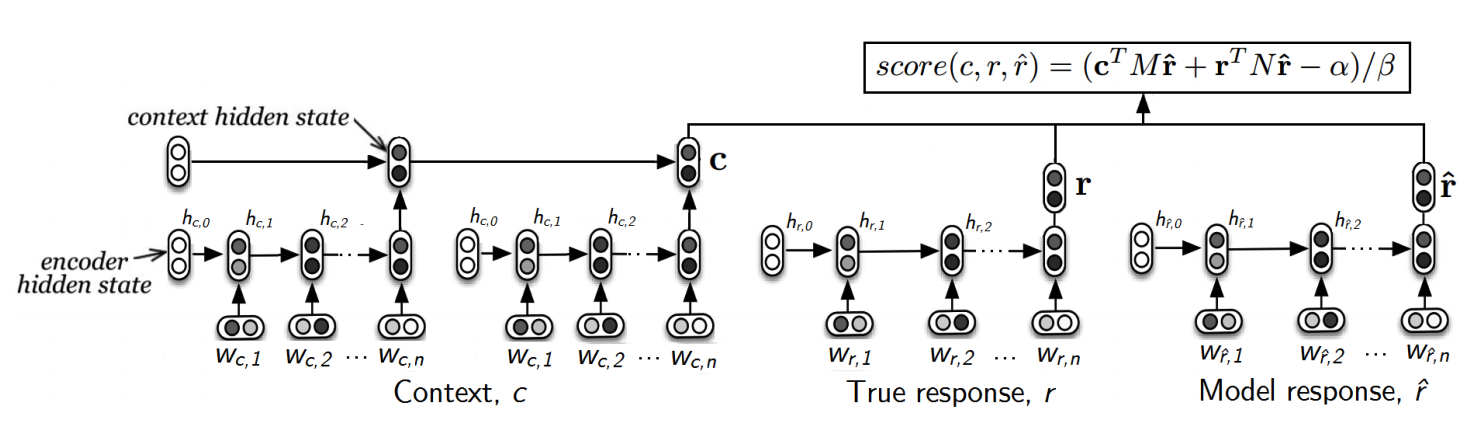$$\mathcal{L}=\sum_{i=1: K}\left[score\left(c_{i}, r_{i}, \hat{r}{i}\right)-h uman{i}\right]^{2}+\gamma|\theta|_{2}$$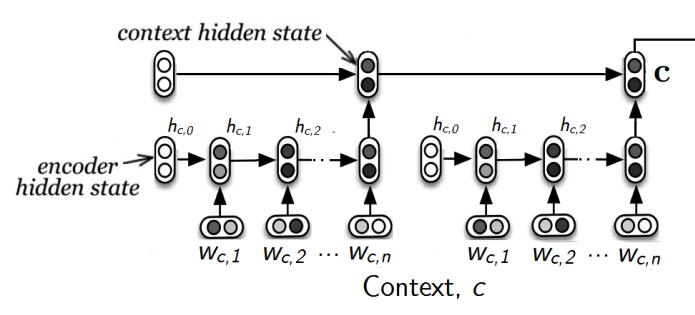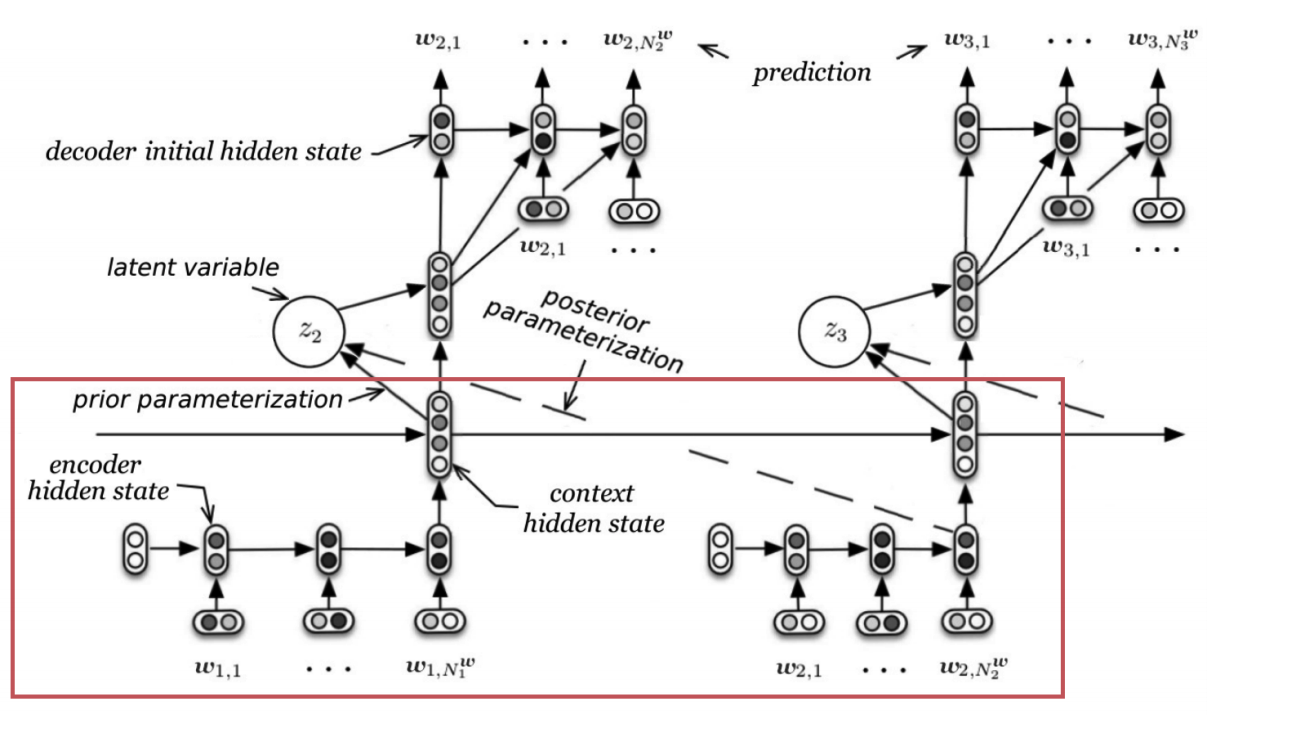5 实验

5.2 结果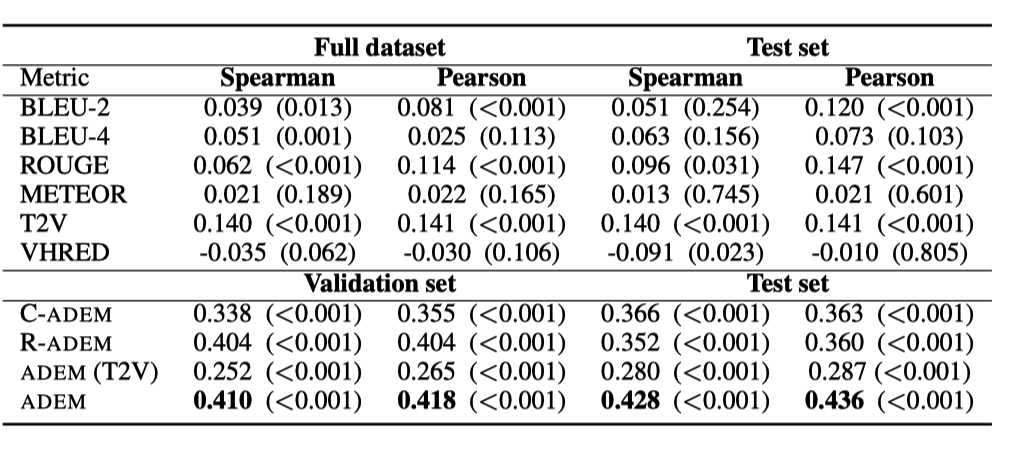Share